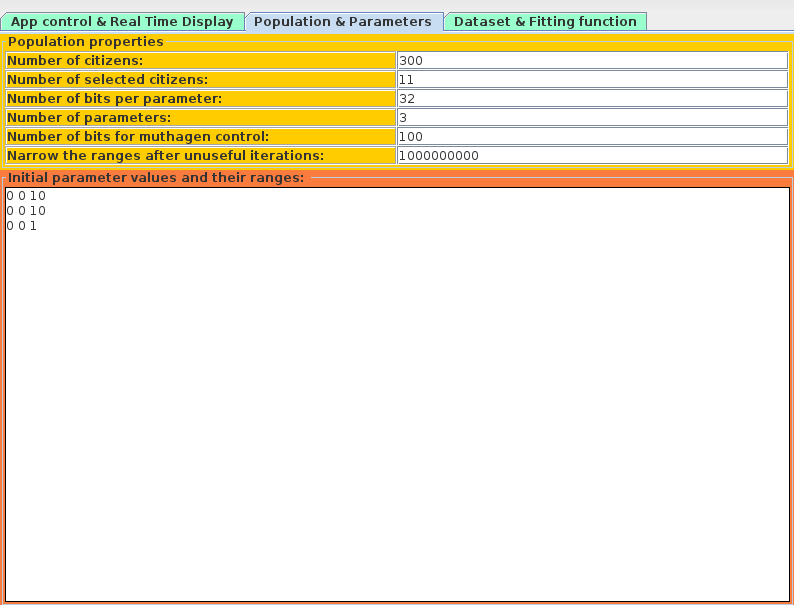##### Personal tools
You are here: Home Acse

# Acse

Acse

AUTHOR: Giuseppe Stanghellini.

ACSE is a program that implements an evolutionary algorithm to optimize a given multiparametric function on a set of points. Its main characteristic is the ease of use.

It is entirely written in Java, it uses a genetic algorithm (GA) to search the minimum difference between a given set of points and the points calculated using a given function in which some parameters have to be determined. The algorithm does the optimization by using a very simple genetic algorithm explained below, the function and its parameters are given by the user, as well as the range of the parameters and their intial values.The algorithm can be summarized as follows:

Each parameter is coded as a binary string of fixed length M, each string representing a value in the range given by the user for that parameter.

Each parameter string is concatenated one after another to form a big string of bits of lenght M * N, where N is the number of parameters of the function.

Each of those big strings is called individual and is considered a genetic representation of a solution, each of them is evaluated with the fitness function, that is the sum of the square of the differences amongst given points and the computed points using the given function. The goal of the algorithm is to find the individual (genetic representation of solution) that minimize the fitting function.The steps implemented by the algorithm are:

1) At the first step of the algorithm an initial population of L individuals is generated randomly.

2) The fitness function for each individual is computed and the K best individuals are choosen and put to the new generation of individuals NG.

3) A new individual is calculated between all couples of those K individuals: a random number between 0 and M * N (number of bits of an individual), called R is generated and then the first R bits from the first individual of the couple and the N * M - R last bits from the second individual of the couple are concatenated to create a new individual. All possible couples are created and from each of them a new individual is generated and added to NG.4) A new set of random individuals is generated and added to NG until the population reaches L number of individuals.

5) The fitness function is evaluated for each individual of the generated population.

6) If the value of the fitting function of the best individual is not below a given threshlod the algortihm returns to step 3.

This algorithm has proven to the author to work quite well, giving consistent solutions where other type of less-esotic algorithm failed, this method has been proved to be less prone to stick into a local (non-optimum) minimum of the fitness function.

The source code along with the compiled JAR can be downloaded here, it is distributed under the GPLv3 license.

A brief explanation of the program can be viewed by clicking on the previous images.

The author of the program can be reached here: Giuseppe Stanghellini.

If you use this program to make scientific works or publications, please cite:
Stanghellini G., 2006, ACSE, http://software.bo.ismar.cnr.it/acse

##### Document Actions